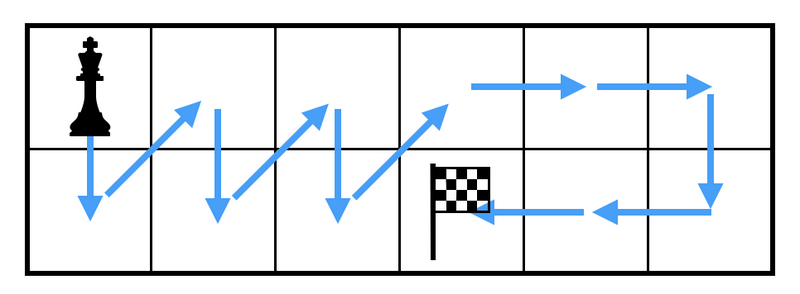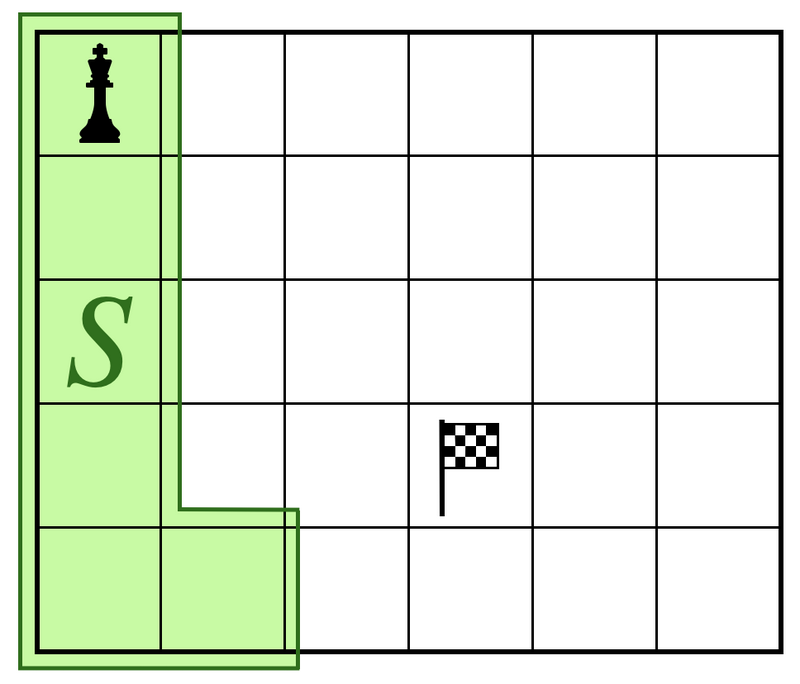Contest Duration: - (local time) (100 minutes) Back to Home

## H - King's Tour Editorial by en_translator

Note that some solution requires complex conditional branches depending on, for example, whether the goal $$(a, b)$$ is on the boundary or not.

Here, in order to avoid complex case divisions, we will try to solve it recursively by boiling down the given problem to that of smaller sizes.

### (1) If $$H = 2$$

We can construct the following tour.Formally, the king is moved as

$$(1, 1) \rightarrow (2, 1) \rightarrow (1, 2) \rightarrow (2, 2) \rightarrow (1, 3) \rightarrow (2, 3) \rightarrow \cdots \rightarrow (1, b-1) \rightarrow (2, b-1) \rightarrow (3-a, b) \rightarrow$$

then as

$$\rightarrow (1, b+1) \rightarrow (1, b+2) \rightarrow \cdots \rightarrow (1, W) \rightarrow (2, W) \rightarrow (2, W-1) \rightarrow \cdots \rightarrow (2, b+1) \rightarrow (a, b)$$.

### (2) If $$H > 2$$ and $$W = 2$$

We can swap the role of rows and columns so that it is boiled down to the “(1) If $$H = 2$$” case above.

### (3) If $$H > 2$$ and $$W>2$$

Divide into two cases by whether the goal $$(a, b)$$ is contained in the region $$S$$ illustrated below.In other words, let

$$S := \lbrace (1, 1), (2, 1) (3, 1), \ldots, (H, 1), (H, 2) \rbrace$$,

and divide into the following cases depending on whether $$(a, b) \in S$$.

#### ・ If $$(a, b) \not\in S$$

If you move the king as > $$(1, 1) \rightarrow (2, 1) \rightarrow (3, 1) \rightarrow \cdots \rightarrow (H, 1) \rightarrow (H, 2)$$, it is then boiled down to the problem of “move the king from $$(H,2)$$ to $$(a,b)$$, visiting every other square.” Therefore, by removing the first column from the board and flipping it vertically, it is boiled down to the problem where $$W$$ is decreased by $$1$$.

#### ・ If $$(a, b) \in S$$

By swapping the roles of row and column, it is boiled down to the “If $$(a, b) \not\in S$$” case above.

A sample code in C++ follows.

#include <iostream>
#include <utility>
#include <vector>
using namespace std;
typedef pair<int, int> P;

vector<P> solve(int h, int w, int a, int b)
{
vector<P> ret;
if(h == 2){
for(int i = 1; i <= b-1; i++) ret.push_back(P(1, i)), ret.push_back(P(2, i));
ret.push_back(P(3-a, b));
for(int i = b+1; i <= w; i++) ret.push_back(P(1, i));
for(int i = w; i >= b+1; i--) ret.push_back(P(2, i));
ret.push_back(P(a, b));
}
else if(h > 2 && w == 2 || b == 1 || a == h && b == 2){
ret = solve(w, h, b, a);
for(auto &p : ret) swap(p.first, p.second);
}
else{
for(int i = 1; i <= h; i++) ret.push_back(P(i, 1));
vector<P> res = solve(h, w-1, h+1-a, b-1);
for(auto &p : res) p.first = h+1-p.first, p.second++;
ret.insert(ret.end(), res.begin(), res.end());
}
return ret;
}

int main(void)
{
int h, w, a, b;
cin >> h >> w >> a >> b;
vector<P> ans = solve(h, w, a, b);
for(auto p : ans) cout << p.first << " " << p.second << endl;
return 0;
}


posted:
last update: